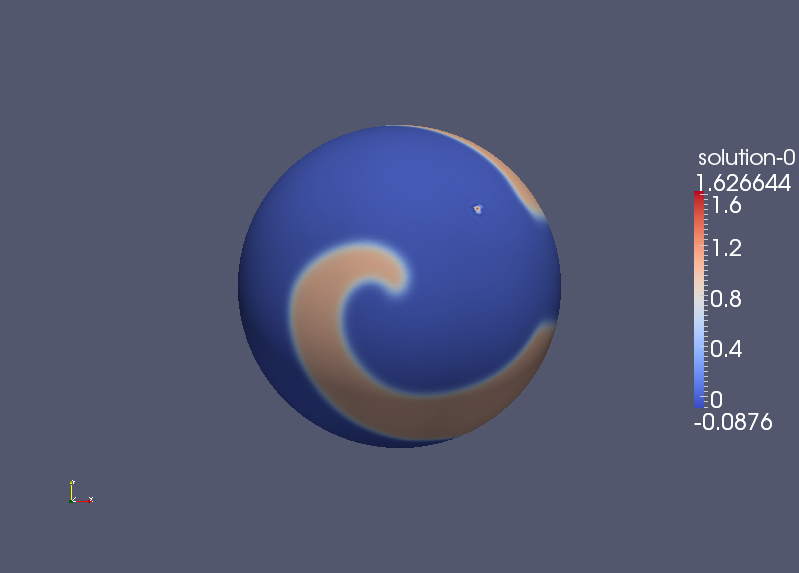# Surfaces with Inhomogeneities

We use the same initial conditions as described in the Fixed Surfaces page for the simulations below.

## The Physical Hole

Here a circular area of the sphere is filtered out and zero Neumann boundary conditions are applied to the hole created. Examples of two holes are given below. The sphere on the left has radius 0.1 and the sphere on the right has radius 0.4.

One can see from the outset that the holes have a pinching effect on the travelling waves, which appears more dramatic for the larger hole. This is the expected behaviour since the waves have an impenetrable barrier ahead of them so they must slow down and travel around the holes. As the simulation runs over time, we noticed that the spiral actually moves closer towards the hole. Eventually, the centre of the spiral will reside in the hole. This is called spiral locking in the literature.

## Reduced Conductivity

Instead of a physical hole, we reduce the conductivity in a region of the sphere to model damaged tissue.

### Zero conductivity

Initially zero conductivity is obtained by setting the diffusion coefficient to zero. The overshoot (the solution be between 0 and 1) in the results below show that there is numerical instability, which in this case is caused by the sudden jump in the value of the diffusion coefficient at the edge of the hole. This problem can be solved in the two ways given below, the first method is to reduce the diffusion coefficent by a factor rather than setting it directly to zero, the second method is to continuously decrease the diffusion coefficient so that it is zero at the centre of the hole.##### Reducing conductivity

As described above, rather than the diffusion coefficent being set to zero here it is reduced by a factor. This has the advantage that as well as the limit being the case of zero conductivity, it is also possible to simulate areas where the tissue is damaged such that its conductivity is reduced but non-zero. The holes simulated are of size 0.01 centered at (0.15,0) where the diffusion coefficient has been reduced by a factor of 100.

##### Continuous conductivity

Here the problem of reducing the difusion coefficent to zero is avoided by continuously decreasing it from the edge of the hole. To this end for a hole of radius $$R$$ centered at $$\mathbf{x_0}$$ the diffusion coefficent is multiplied by a factor

\begin{equation*} 1+ \exp \left( 1000 \left( \left| \mathbf {x}-\mathbf{ x_0} \right|^2 -R^2 \right) \right) \exp \left( {{-R} \over {10 }} \right) - \exp \left( {{- \left| \mathbf{x}- \mathbf{x_0} \right|^2 } \over {10R }} \right). \end{equation*}

Putting aside the middle term for the moment, the sum is

\begin{equation} 1- \exp \left( {{- \left| \mathbf{x}-\mathbf{ x_0 }\right|^2 } \over {10R }} \right), \label{eq:fac} \end{equation}

which decays like a Gaussian with variance $$\sigma^2 =5R$$ to zero in the center of the hole. The term

\begin{equation*} \exp \left( {{-R} \over {10}} \right) \end{equation*}

is the difference between the sum of the first and third term of our original factor and is one at the edge of the hole. This is multplied by a Gaussian so that the contribution decays very rapidly away from the hole. Thus we have a continuous function that sets the diffusion factor to zero at the center of the hole and is one at the edges of the hole. Again our test case uses a circular hole. The left hand hole is centered at $$( 0.3,0.3, \sqrt {0.82} )$$ and has radius $$R=0.1$$, and the right hand hole is centred at $$(0.3,-0.3 , \sqrt{0.82} )$$ with radius $$R=0.2$$.